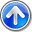# The ZhemweL

Seputar Dunia IT dan Fakta Unik Lainnya

## Saturday, 23 July 2016

### 16.1 Production of Resin-Containing Panels

There exists a variety of synthetic panels for both interior and exterior use. Typical applications are ofﬁce desktops and facade cladding. One type of panel is made of polymerised resin reinforced with wood ﬁbres or sulphate paper.

In manufacturing these panels we can distinguish two stages. In the ﬁrst stage sheets of resin-impregnated paper enclosed by two layers of padded paper are pressed together. Duringthispressingstagethecomponentsarecompressedtowithin10percentoftheirﬁnal thickness. A schematic three-layer model of a panel is given in Figure 16.1. A panel thus has two bolsters of padded paper and a core consisting of impregnated paper. In the second stage high temperatures are applied at the boundaries of the panel, which causes heating of the panel. This induces a polymerisation reaction in the core. Heat is released during the polymerisation, which again leads to an increase of temperature in the panel. This process is called resin curing and continues until the chemicals in the core are depleted. When the polymerisation reaction is ﬁnished, the temperature ﬁnally tends to a steady state. In this section we introduce a model for the resin-curing process.

#### 16.1.1 Modeling the Curing Process

ThecuringprocesscanbedescribedbythetemperatureT inthepanelandtheconcentration C of chemicals involved in the polymerisation reaction in the core. To keep the model feasible we make the following assumptions :

1. The materials are incompressible.
2. Resin curing is a ﬁrst order reaction.
3. Diffusion of resin is negligible.
4. All variables depend only on the transverse space coordinate x.

The governing equations are the following. In both bolsters the heat equation holds, which in our particular case is given by 

with ρ, cp, and λ the density, speciﬁc heat, and thermal conductivity, respectively, of the bolstermaterial. Theseparametersareassumedtobeconstant. Inthecore,heatconduction is coupled with the polymerisation reaction, and the governing equations read

with ^H and k the enthalpy of polymerisation and the reaction rate, respectively. We assume also that the parameters ρ, cp, λ, and ^H in (16.2a) and (16.2b) are constant. The reaction rate is given by theArrhenius expression

with A, Ea, and R the preexponential factor, the activation energy, and the universal gas constant, respectively. The reaction rate k as a function of the temperature T is shown in Figure 16.2. The values of all physical parameters/constants are given in Table 16.1.

Since the parameters ρ, cp, λ, and ^H are constant, the heat equations (16.1) and (16.2a) can be simpliﬁed. Introducing the thermal conductivity a and the coefﬁcient b of temperature increase per unit concentration decrease, in short the temperature coefﬁcient, by

in the core. Equation (16.6) is coupled with (16.2b) for the concentration.

The model (16.5), (16.6), and (16.2b) has to be completed with initial and boundary conditions and conditions at the interfaces between core and bolsters. As initial conditions, we choose a constant temperature and concentration :

These conditions mean that there is no accumulation of heat at the interfaces. Note that we do not have interface conditions for the concentration C and that we do not need them either.

and depends strongly on the temperature. Heat conduction and the polymerisation reaction are essentially coupled when both time scales are of the same order of magnitude. This is the case when the order of magnitude of the temperature is as follows :

#### 16.1.2 Numerical Solution Method

Inordertocomputeanumericalsolutionoftheinitialboundaryvalueproblem(16.5),(16.6), (16.2b), and (16.7) to (16.10), we introduce the spatial grid

The coefﬁcient dr in (16.16) is deﬁned by

and might be called the conduction number. Likewise, for the equations (16.6) and (16.2b) in the core we have

The coefﬁcient d2 in (16.18a) is deﬁned in (16.17). Finally, at the interfaces the following discrete equations hold :

#### 16.1.3 Discussion and Related Problems

• The proposed model is rather elementary. In the real resin-curing process more complex chemistry, involving more chemical species and reactions, should be taken into account. Also the diffusion of all species should be modeled. Finally, the onedimensional approximation is most likely not accurate near the ends of the panel. A three-dimensional model should be used instead.
• Amoredetaileddescriptionofresin-containingpanels,includingdeformationthrough moisture transport, is given in [54, 23].
• The resin-curing process is an example of a reaction-diffusion problem. These problemsoccurinmanyﬁeldsofscienceandengineering,e.g.,inmodelsforﬂamepropagation,biochemicalreactions,andpopulationdynamics. Examplesinthistextarethe thermalexplosioninavessel(Section16.3)andthecatalyzerpelletproblem(Section 16.8). Moreover, we discussed Fisher’s equation, which is a model problem from population dynamics, in Section 10.6.2.